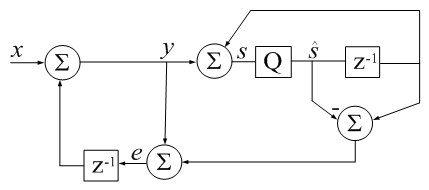Accumulator Error Feedback

(Difference between revisions)
 Revision as of 21:46, 28 November 2017 (edit) (Undo revision 3133 by Ranjelin (Talk))← Previous diff Revision as of 21:49, 28 November 2017 (edit) (undo)Next diff → Line 1: Line 1: [[Image:Gleich.jpg|thumb|right|429px|CSUM() in Digital Signal Processing terms: [[Image:Gleich.jpg|thumb|right|429px|CSUM() in Digital Signal Processing terms: z-1 is a unit delay, Q is a floating-point quantizer to 64 bits, z-1 is a unit delay, Q is a floating-point quantizer to 64 bits, - qi represents error due to quantization (additive by definition).
-Jon Dattorro]] + qi represents error due to quantization (additive by definition).   -[/itex] Jon Dattorro]]

function s_hat = csum(x)                                                                                                                                            function s_hat = csum(x)

Revision as of 21:49, 28 November 2017CSUM() in Digital Signal Processing terms: z-1 is a unit delay, Q is a floating-point quantizer to 64 bits, qi represents error due to quantization (additive by definition).$LaTeX: -$ Jon Dattorro
function s_hat = csum(x)
% CSUM Sum of elements using a compensated summation algorithm.
%
% For large vectors, the native sum command in Matlab does
% not appear to use a compensated summation algorithm which
% can cause significant roundoff errors.
%
% This code implements a variant of Kahan's compensated
% summation algorithm which often takes about twice as long,
% but produces more accurate sums when the number of
% elements is large. -David Gleich
%
% Also see SUM.
%
% % Matlab csum() example:
% clear all
% csumv = 0;
% while ~csumv
%    v = randn(13e6,1);
%    rsumv = abs(sum(v) - sum(v(end:-1:1)));
%    disp(['rsumv = ' num2str(rsumv,'%18.16f')]);
%    [~, idx] = sort(abs(v),'descend');
%    x = v(idx);
%    csumv = abs(csum(x) - csum(x(end:-1:1)));
%    disp(['csumv = ' num2str(csumv,'%18.16e')]);
% end

s_hat=0; e=0;
for i=1:numel(x)
s_hat_old = s_hat;
y = x(i) + e;
s_hat = s_hat_old + y;
e = (s_hat_old - s_hat) + y;  %calculate difference first (Higham)
end
return